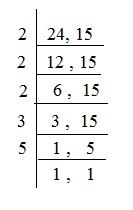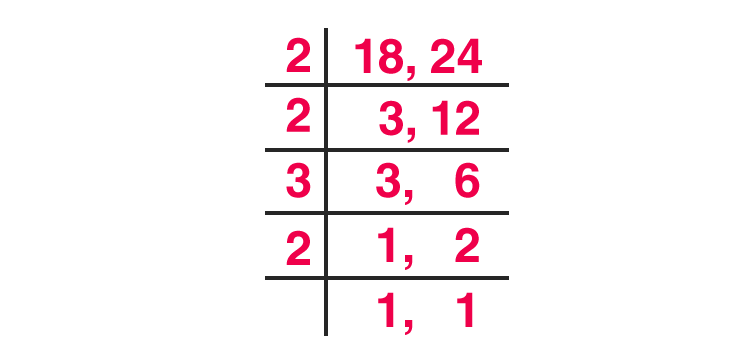# LCM of Two Numbers

The LCM of two numbers is the smallest number which divides the 2 numbers evenly. To find the LCM of two numbers, it is important to check whether the denominators of the two numbers are common or uncommon. Let’s find out how to find the least common multiple i.e. of any two numbers with the same or different denominators and also, the LCM of fractions.

## What is LCM of 2 Numbers?

The LCM of Least Common Multiple of 2 numbers is the smallest number which can divide the two numbers equally. It is is also known as the Least Common Divisor or LCD. For example, consider 2 numbers as 10 and 4. Now, the smallest number that can divide 10 and 4 evenly will be 20.

## How to Find LCM of Two Numbers?

There are 4 main methods to calculate the least common multiple of 2 numbers. These methods are:

• Listing Multiples or Brute Force Method
• Prime Factorization Method
• Division Method or Ladder Method
• GCD or GCF Method
 Try This: LCM Calculator of Two Numbers

### Listing Multiples or Brute Force Method of Finding LCM

In this method, the multiples of each number are listed until the first common multiple is found. Consider the example of 4 and 10. For this method, the multiples of 4 and 10 are to be listed.

Multiples of 4: 4, 8, 12, 16, 20, 24, 28

Multiples of 10: 10, 20, 30, 40

Here, the number 20 is the first common multiple of both 4 and 10. So, the LCM of 4, 10 is 20.

### Prime Factorization Method of Finding LCM

The prime factorization is one of the most common ways of finding LCM. To find the LCM of two numbers 30 and 45, the steps are as follows:

Step 1: To first list the prime factors of each number.

30 = 2 × 3 × 5
45 = 3 × 3 × 5

Step 2: Next multiply each factor the maximum number of times it occurs in either number.

If the same factor occurs more than once in both numbers, then multiply the factor the maximum number of times it occurs.

The occurrence of Numbers in the above example:

2: one time
3: two times
5: one time
LCM = 2 × 3 × 3 × 5 = 90

After calculating the LCM, always check to be sure your answer can be divided evenly by both numbers.

### Division Method or Ladder Method of Finding LCM

In this method, the two numbers are simultaneously divided with prime numbers until the division is even. When there are no more primes that evenly divide into both numbers, multiply the divisors to get the LCM. For example, consider 4 and 10 as twoDivision Method or Ladder Method of Finding LCM

Here, the LCM of 24, 15 will be 2×2×2×3×5=23×3×5=120

### GCD or GCF Method of Finding LCM

This method is used only when the greatest common factor of two numbers is given. The formula used to find the LCM using the GCF or GCD is:

L.C.M. = a×b/ gcd(a,b)

For example, for 15 and 24, the GCF will be 3. So, the LCM will be (15 × 24) / 3 = 3.

## Example Problems for LCM of two numbers

Example 1: Find the L.C.M of 18 and 24 by using the division method?

Solution:For numbers 18 and 24 = 2 × 2 × 3 × 2 = 24 is the LCM.

Example 2: Find the Least Common Multiples of these sets of numbers – 3, 9, 21

Solution:

Step 1: List the prime factors of each.
3: 3
9: 3 × 3
21: 3 × 7

Step 2: Multiply each factor the maximum number of times it occurs in any of the numbers.

The occurrence of Numbers in the above example:

3: two times

7: one time

3 x 3 x 7 = 63

9 has two 3s, and 21 has one 7, so we multiply 3 two times, and 7 once.

This gives us 63, the lowest number that can be divided evenly by 3, 9, and 21.

Example 3: Find the Least common factor of 12, 80.

Solution:

Step 1: List the prime factors of each.
12: 2 × 2 × 3
80: 2 × 2 × 2 × 2 × 5

Step 2:
Multiply each factor the maximum number of times it occurs in either number.

Step 3:

The occurrence of Numbers in the above example:

2: 4 times

3: 1 time

5: 1 time

2 x 2 x 2 x 2 x 3 x 5 =240

12 has one 3, and 80 has four 2’s and one 5, so we multiply 2 four times, 3 once, and five once.

This gives us 240, the lowest number that can be divided by both 12 and 80.

## How to Find LCM of Fractions?

Formula to find the LCM of two fractions is:

 L.C.M = $\frac{LCM\, of\, the\, Numerators}{HCF\, of\, the\, Denominators}$
• Step 1: If the two fractions are :
$\frac{a}{b}$ and $\frac{c}{d}$
• Step 2: LCM of two numbers is the lowest/smallest number which is a multiple of both.

HCF of two numbers is the largest/maximum whole number which is a factor of both.

• Step 3: Let’s learn it using an example:

LCM of ⅘ and 3/7

LCM of the numbers, $\frac{4}{3}$ / $\frac{5}{7}$ = LCM

12/1 = 12

HCF of the numbers, $\frac{4}{3}$/$\frac{5}{7}$ = HCF

1/35

L.C.M = LCM of the numerators/HCF of denominators

L.C.M = $\frac{12}{1/35}$

L.C.M = 12 x 35 = 420.

Register to BYJU’S to understand more mathematical topics with a detailed explanation and more practice examples.